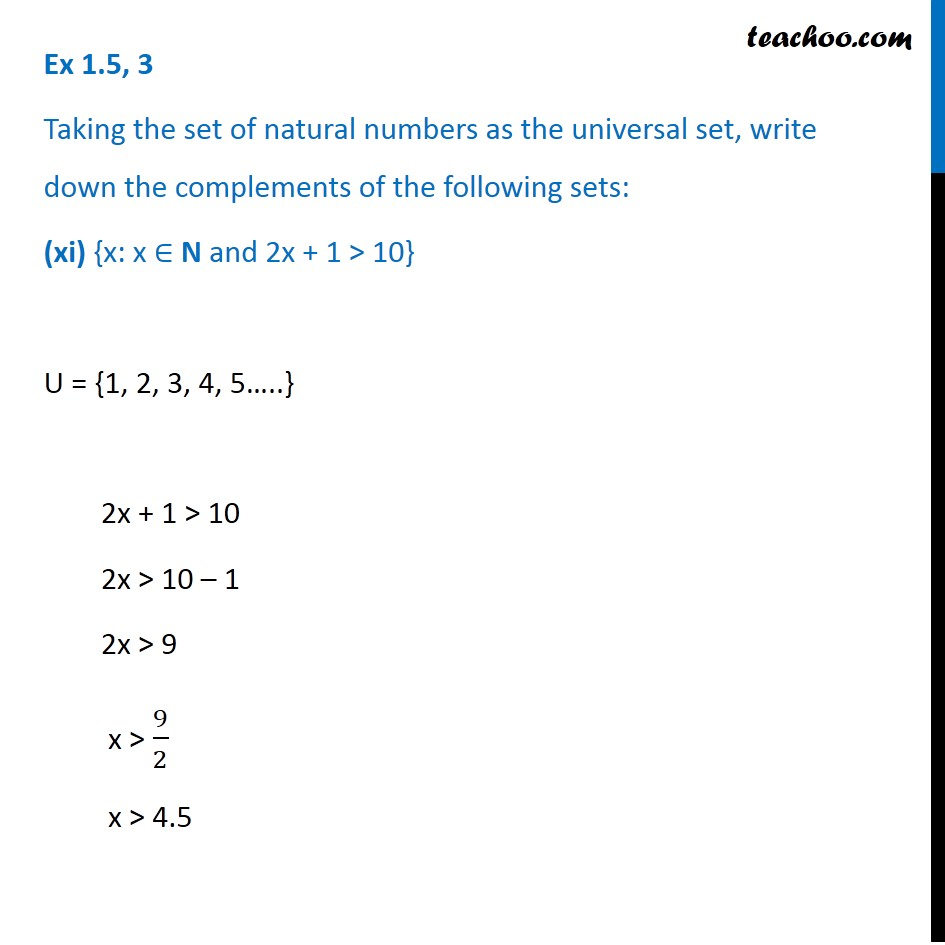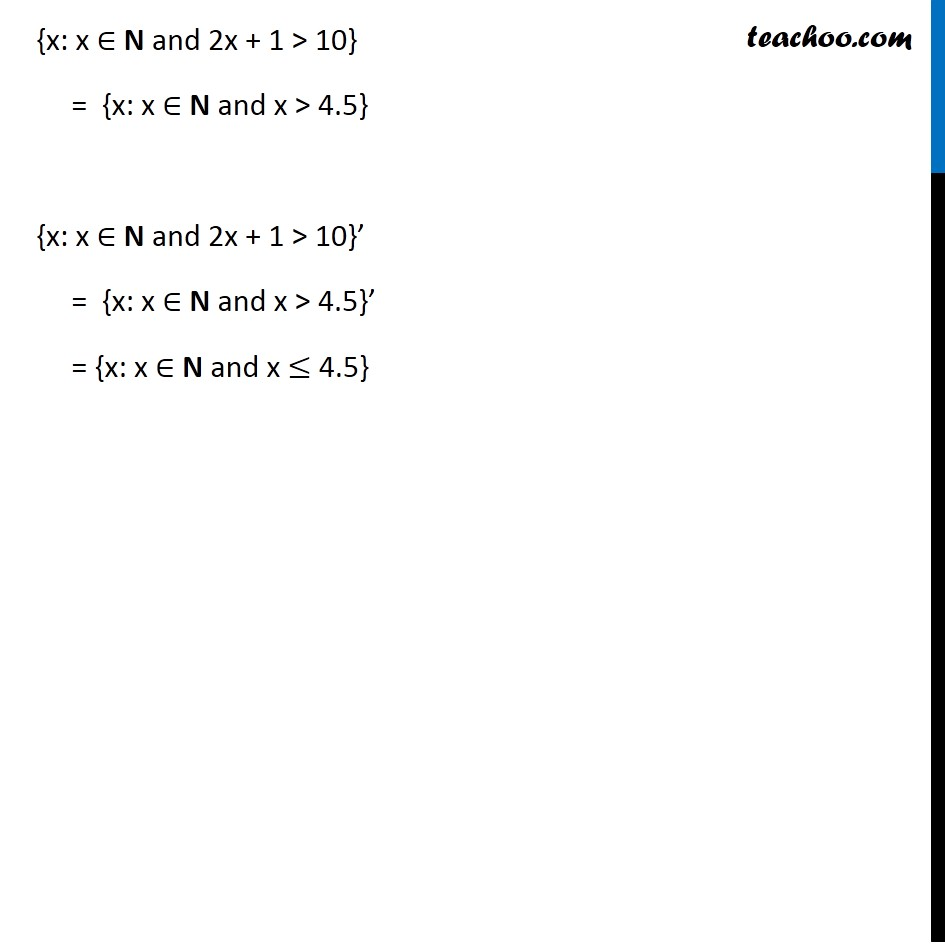Ex 1.5

Chapter 1 Class 11 Sets
Serial order wiseLearn in your speed, with individual attention - Teachoo Maths 1-on-1 Class

### Transcript

Ex 1.5, 3 Taking the set of natural numbers as the universal set, write down the complements of the following sets: (xi) {x: x ∈ N and 2x + 1 > 10} U = {1, 2, 3, 4, 5…..} 2x + 1 > 10 2x > 10 – 1 2x > 9 x > 9/2 x > 4.5 {x: x ∈ N and 2x + 1 > 10} = {x: x ∈ N and x > 4.5} {x: x ∈ N and 2x + 1 > 10}’ = {x: x ∈ N and x > 4.5}’ = {x: x ∈ N and x ≤ 4.5}